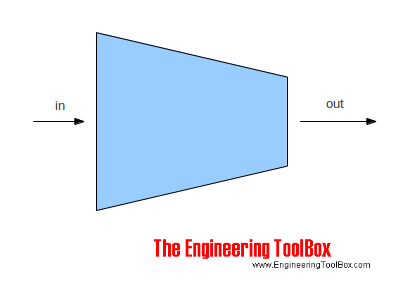Engineering ToolBox - Resources, Tools and Basic Information for Engineering and Design of Technical Applications!

# Fluid Flow - Equation of Continuity

## The Equation of Continuity is a statement of mass conservation.

The Law of Conservation of Mass states that mass can be neither created or destroyed. Using the Mass Conservation Law on a steady flow process - flow where the flow rate do not change over time - through a control volume where the stored mass in the control volume do not change - implements that

• inflow equals outflow

This statement is called the Equation of Continuity. Common application where the Equation of Continuity are used are pipes, tubes and ducts with flowing fluids or gases, rivers, overall processes as power plants, diaries, logistics in general, roads, computer networks and semiconductor technology and more.The Equation of Continuity and can be expressed as:

m = ρi1 vi1 Ai1 + ρi2 vi2 Ai2 + .... + ρin vin Ain

= ρo1 vo1 Ao1 + ρo2 vo2 Ao2 + .... + ρom vom Aom                            (1)

where

m = mass flow rate (kg/s)

ρ = density (kg/m3)

v = speed (m/s)

A = area (m2)

With uniform density equation (1) can be modified to

q = vi1 Ai1 + vi2 Ai2 + .... + vin Ain

= vo1 Ao1 + vo2 Ao2 + .... + vom Aom                         (2)

where

q = flow rate (m3/s)

ρi1 = ρi2 = . . = ρin = ρo1 = ρo2 = .... = ρom

For a simple reduction (or expansion) as indicated in the figure above - the equation of continuity for uniform density can be transformed to

vin Ain = vout Aout                             (3)

or

vout = vin Ain / Aout                            (3b)

### Example - Equation of Continuity

10 m3/h of water flows through a pipe with 100 mm inside diameter. The pipe is reduced to an inside dimension of 80 mm.

Using equation (2) the velocity in the 100 mm pipe can be calculated

(10 m3/h) (1 / 3600 h/s) = v100 (3.14 (0.1 m)2 / 4)

or

v100 = (10 m3/h) (1 / 3600 h/s) / (3.14 (0.1 m)2 / 4)

= 0.35 m/s

Using equation (2) the velocity in the 80 mm pipe can be calculated

(10 m3/h) (1 / 3600 h/s) = v80 (3.14 (0.08 m)2 / 4)

or

v80 = (10 m3/h) (1 / 3600 h/s) / (3.14 (0.08 m)2 / 4)

= 0.55 m/s

### Equation of Continuity Calculator

The calculator below is based on eq. 3b and can be used for fluids with uniform density - typical liquids like water, oil and more.

vin - input velocity (m/s, fpm)

Ain - input area (m2, sq.ft)

Aout - output area (m2, sq.ft)

## Related Topics

• ### Dimensions

Sizes and dimensions of pipes and tubes, and their fittings - inside and outside diameter, weight and more.
• ### Fluid Flow and Pressure Loss

Pipe lines - fluid flow and pressure loss - water, sewer, steel pipes, pvc pipes, copper tubes and more.
• ### Fluid Mechanics

The study of fluids - liquids and gases. Involving velocity, pressure, density and temperature as functions of space and time.

## Related Documents

• ### Bernoulli Equation

Conservation of energy in a non-viscous, incompressible fluid at steady flow.
• ### Conservation of Mass

The Law of Conservation of Mass states that mass can neither be created or destroyed.
• ### Ducts Sizing - the Velocity Reduction Method

The velocity reduction method can be used when sizing air ducts.
• ### Equations in Fluid Mechanics

Equations used in fluid mechanics - like Bernoulli, conservation of energy, conservation of mass, pressure, Navier-Stokes, ideal gas law, Euler equations, Laplace equations, Darcy-Weisbach Equation and more.
• ### Mechanical Energy Equation vs. Bernoulli Equation

The Mechanical Energy Equation compared to the Extended Bernoulli Equation.
• ### Orifice, Nozzle and Venturi Flow Rate Meters

The orifice, nozzle and venturi flow rate meters makes the use of the Bernoulli Equation to calculate fluid flow rate using pressure difference through obstructions in the flow.
• ### Sluice Gate - Volume Flow Measurements

Sluice gates can be used to control and measure volume flow rates in open channels and rivers, mainly in connection to hydro power plants.
• ### Technical Terms in Fluid Mechanics

Some commonly used technical terms in fluid mechanics.

## Engineering ToolBox - SketchUp Extension - Online 3D modeling!

Add standard and customized parametric components - like flange beams, lumbers, piping, stairs and more - to your Sketchup model with the Engineering ToolBox - SketchUp Extension - enabled for use with older versions of the amazing SketchUp Make and the newer "up to date" SketchUp Pro . Add the Engineering ToolBox extension to your SketchUp Make/Pro from the Extension Warehouse !

We don't collect information from our users. More about

## Citation

• The Engineering ToolBox (2003). Fluid Flow - Equation of Continuity. [online] Available at: https://www.engineeringtoolbox.com/equation-continuity-d_180.html [Accessed Day Month Year].

Modify the access date according your visit.

9.19.12